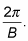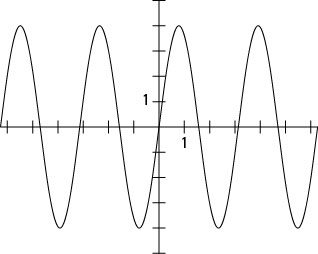##### Trigonometry Workbook For DummiesA general equation for the sine function is y = A sin Bx. The A and B are numbers that affect the amplitude and period of the basic sine function, respectively.

The graph of the function y = A sin Bx has an amplitude of A and a period ofThe amplitude, A, is the distance measured from the y-value of a horizontal line drawn through the middle of the graph (or the average value) to the y-value of the highest point of the sine curve, and B is the number of times the sine curve repeats itself within 2π, or 360 degrees.

By keeping these two values in mind, you can quickly sketch the graph of a sine curve — or picture it in your head. For example, when graphing y = 4sin 2x, you would follow these steps:

The amplitude is 4, so the curve will extend up 4 units and down 4 units from the middle. To allow for some space above and below, set the y-axis to go from –5 to 5.

2. Take into account the period.

Two complete graphs of the sine are within the space that usually houses only one.

3. Graph the curve from –2π to 2π (see the following figure).The graph of y = 4sin 2x.

You can see that the graph goes from –4 to 4 and that four complete cycles are in the space that usually houses only two.